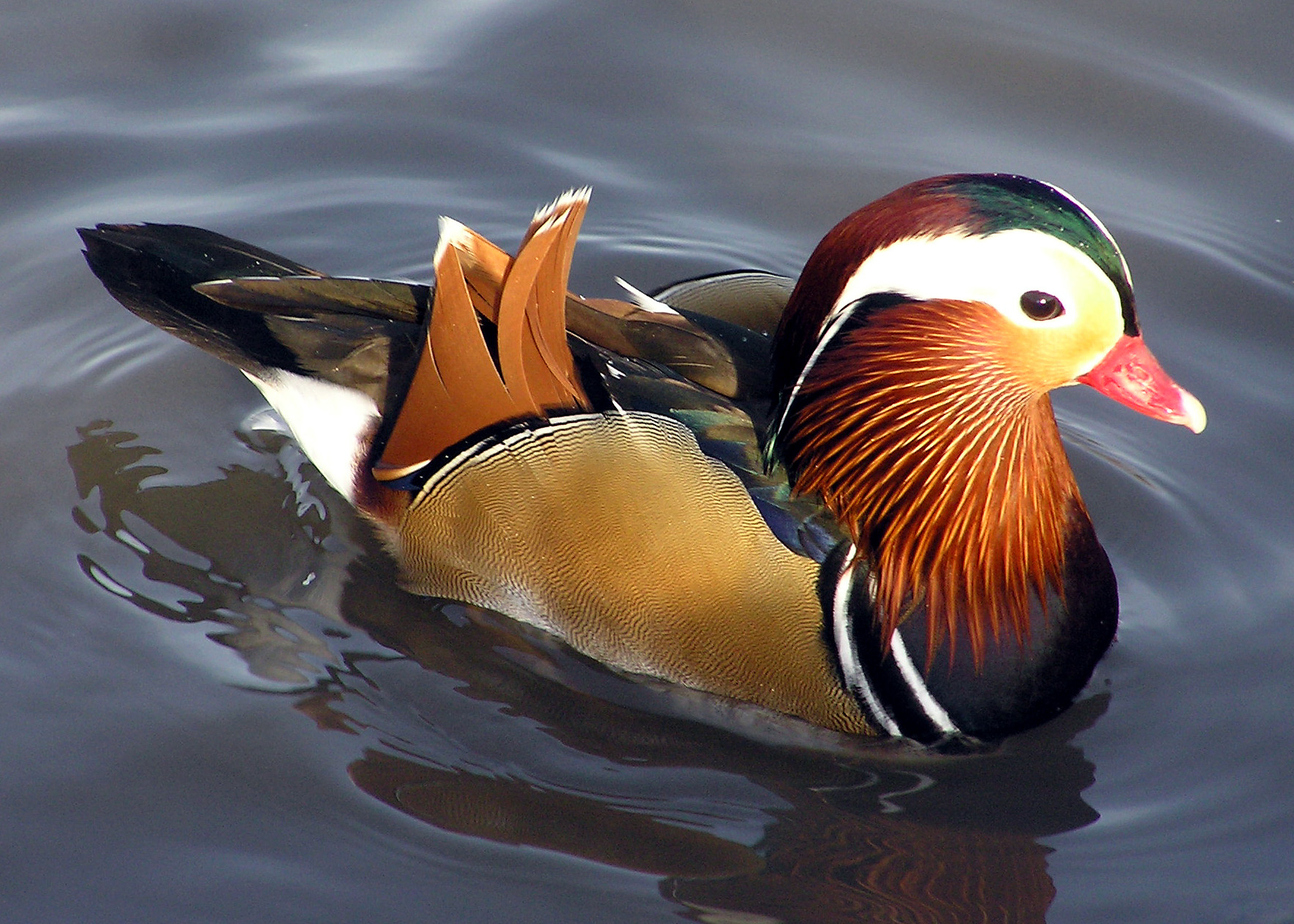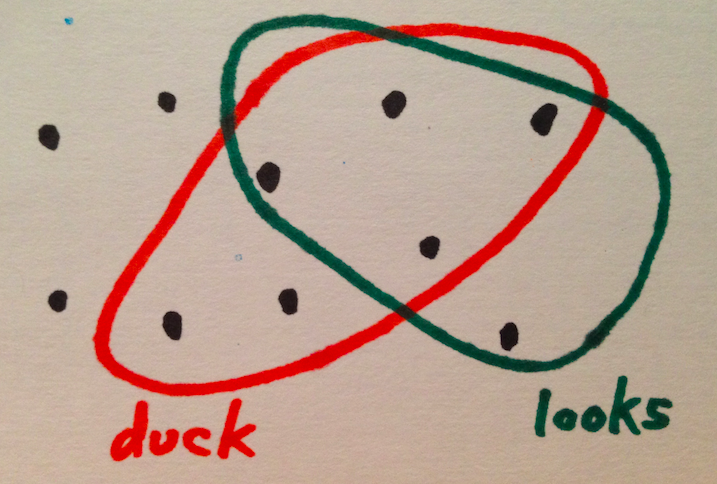# Bayes' Rule for Ducks

Sunday February 23, 2014

You look at a thing.Is it a duck?

Re-phrase: What is the probability that it's a duck, if it looks like that?

Bayes' rule says that the probability of it being a duck, if it looks like that, is the same as the probability of any old thing being a duck, times the probability of a duck looking like that, divided by the probability of a thing looking like that.

$Pr(duck | looks) = \frac{Pr(duck) \cdot Pr(looks | duck)}{Pr(looks)}$

This makes sense:

• If ducks are mythical beasts, then $$Pr(duck)$$ (our "prior" on ducks) is very low, and the thing would have to be very duck-like before we'd believe it's a duck. On the other hand, if we're at some sort of duck farm, then $$Pr(duck)$$ is high and anything that looks even a little like a duck is probably a duck.
• If it's very likely that a duck would look like that ($$Pr(looks|duck)$$ is high) then we're more likely to think it's a duck. This is the "likelihood" of a duck looking like that thing. In practice it's based on how the ducks we've seen before have looked.
• The denominator $$Pr(looks)$$ normalizes things. After all, we're in some sense portioning out the probabilities of this thing being whatever it could be. If 1% of things look like this, and 1% of things look like this and are ducks, then 100% of things that look like this are ducks. So $$Pr(looks)$$ is what we're working with; it's the denominator.

Here's an example of a strange world to test this in:There are ten things. Six of them are ducks. Five of them look like ducks. Four of them both look like ducks and are ducks. One thing looks like a duck but is not a duck. Maybe it's a fake duck? Two ducks do not look like ducks. Ducks in camouflage. Test the equality of the two sides of Bayes' rule:

$Pr(duck | looks) = \frac{Pr(duck) \cdot Pr(looks | duck)}{Pr(looks)}$

$\frac{4}{5} = \frac{\frac{6}{10} \cdot \frac{4}{6}}{\frac{5}{10}}$

It's true here, and it's not hard to show that it must be true, using two ways of expressing the probability of being a duck and looking like a duck. We have both of these:

$Pr(duck \cap looks) = Pr(duck|looks) \cdot Pr(looks)$

$\displaystyle Pr(duck \cap looks) = Pr(looks|duck) \cdot Pr(duck)$

Check those with the example as well, if you like. Using the equality, we get:

$Pr(duck|looks) \cdot Pr(looks) = Pr(looks|duck) \cdot Pr(duck)$

Then dividing by $$Pr(looks)$$ we have Bayes' rule, as above.

$Pr(duck | looks) = \frac{Pr(duck) \cdot Pr(looks | duck)}{Pr(looks)}$

This is not a difficult proof at all, but for many people the result feels very unintuitive. I've tried to explain it once before in the context of statistical claims. Of course there's a wikipedia page and many other resources. I wanted to try to do it with a unifying simple example that makes the equations easy to parse, and this is what I've come up with.

This post was originally hosted elsewhere.